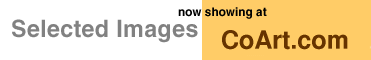[ Graphics Archive | Up | Comments ]

### Special Topics:Computational Geometry

Delaunay triangulation with Qhull by Brad BarberThis picture shows how the Delaunay triangulation (in light green) is derived from a convex hull (the 3-d object). Each vertex of the triangulation corresponds to a vertex of the convex hull. Each edge of the triangulation corresponds to an edge of the convex hull.

The Delaunay triangulation is the triangulation with empty circumcircles for each triangle. The dual of the Delaunay triangulation is the Voronoi diagram. Both structures have many applications in science, engineering, and mathematics.

To compute the Delaunay triangulation, project the points to a paraboloid by summing the squares of their coordinates. Then take the convex hull of the projected points. Remove the vertical dimension from the lower envelope of the convex hull. The projected edges are the Delaunay triangulation of the original points.

Notice the 6 co-circular points near the center of the triangulation. They correspond to a flat facet of the convex hull. The program Qhull constructed a hexagon instead of three triangles. The hexagon has an empty circumcircle.

The same process can be used for points in 3-d or higher. In 3-d, each tetrahedron of the Delaunay triangulation has an empty circumsphere, and the corresponding paraboloid sits in 4-d.

How to make it: `qhull < eg.data.17 C-0 d Ga >a (q_eg in qhull)`

Image created: April 1995The Geometry Center Home Page